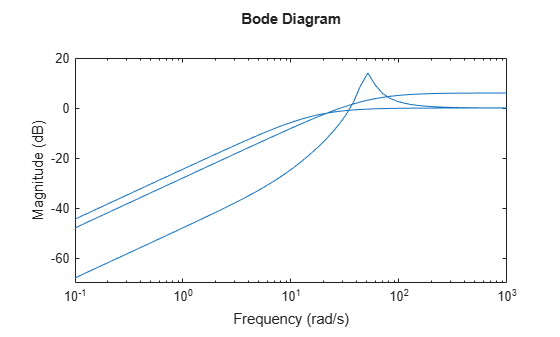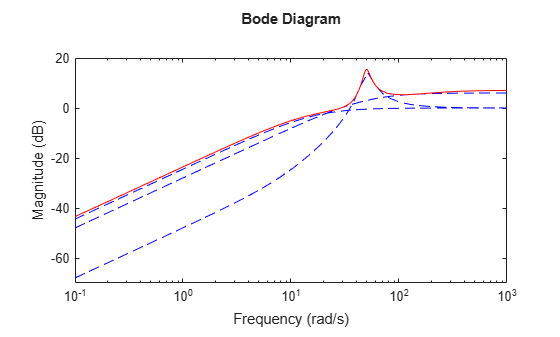Documentation

# ucover

Fit an uncertain model to set of LTI responses

## Syntax

```usys = ucover(Parray,Pnom,ord) usys = ucover(Parray,Pnom,ord1,ord2,utype) [usys,info] = ucover(Parray,...) [usys_new,info_new] = ucover(Pnom,info,ord1_new,ord2_new) ```

## Description

`usys = ucover(Parray,Pnom,ord)` returns an uncertain model `usys` with nominal value `Pnom` and whose range of behaviors includes all responses in the LTI array `Parray`. The uncertain model structure is of the form $usys=Pnom\left(1+W\left(s\right)\Delta \left(s\right)\right)$, where

• Δ is an `ultidyn` object that represents uncertain dynamics with unit peak gain.

• W is a stable, minimum-phase shaping filter that adjusts the amount of uncertainty at each frequency.

`ord` is the number of states (order) of W```. Pnom``` and `Parray` can be `ss`, `tf`, `zpk`, or `zpk` models. `usys` is of class `ufrd` when `Pnom` is an `frd` model and is an `uss` model otherwise.

`usys = ucover(Parray,Pnom,ord1,ord2,utype)` specifies the order `ord1` and `ord2` of each diagonal entry of W1 and W2, where W1 and W2 are diagonal, stable, minimum-phase shaping filters. `utype` specifies the uncertain model structure, as described in Uncertain Model Structures, and can be '`InputMult`' (default), '`OutputMult`' or '`Additive`'. `ord1` and `ord2` can be:

• `[]`, which implies that the corresponding filter is 1.

• Scalar, which implies that the corresponding filter is scalar-valued.

• Vectors with as many entries as diagonal entries in W1 and W2.

`[usys,info] = ucover(Parray,...)` returns a structure `info` that contains optimization information. `info.W1opt` and` Info.W2opt` contain the values of W1 and W2 computed on a frequency grid and `info.W1` and` info.W2` contain the fitted shaping filters W1 and W2.

`[usys_new,info_new] = ucover(Pnom,info,ord1_new,ord2_new)` improves the fit using initial filter values in `info and` new orders `ord1_new` and `ord2_new` of W1 and W2. This syntax speeds up command execution by reusing previously computed information. Use this syntax when you are changing filter orders in an iterative workflow.

## Examples

collapse all

Fit an uncertain model to an array of LTI responses. The responses might be, for example, the results of multiple runs of acquisition of frequency response data from a physical system.

For the purpose of this example, generate the frequency response data by creating an array of LTI models and sampling the frequency response of those models.

```Pnom = tf(2,[1 -2]); p1 = Pnom*tf(1,[.06 1]); p2 = Pnom*tf([-.02 1],[.02 1]); p3 = Pnom*tf(50^2,[1 2*.1*50 50^2]); array = stack(1,p1,p2,p3); Parray = frd(array,logspace(-1,3,60));```

The frequency response data in `Parray` represents three separate data acquisition experiments on the system.

Plot relative errors between the nominal plant response and the three models in the LTI array.

```relerr = (Pnom-Parray)/Pnom; bodemag(relerr)```If you use a multiplicative uncertainty model structure, the magnitude of the shaping filter should fit the maximum relative errors at each frequency.

Try a 1st-order shaping filter to fit the maximum relative errors.

`[P,Info] = ucover(Parray,Pnom,1);`

`P` is an uncertain state-space (`uss`) model that captures the uncertainty as a `ultidyn` uncertain dynamics block.

`P.Uncertainty`
```ans = struct with fields: Parray_InputMultDelta: [1x1 ultidyn] ```

The `Info` structure contains other information about the fit, including the shaping filter. Plot the response to see how well the shaping filter fits the relative errors.

`bodemag(relerr,'b--',Info.W1,'r',{0.1,1000});`The plot shows that the filter `W1` is too conservative and exceeds the maximum relative error at most frequencies. To obtain a tighter fit, rerun the function using a 4th-order filter.

`[P,Info] = ucover(Parray,Pnom,4);`

Evaluate the fit by plotting the Bode magnitude plot.

`bodemag(relerr,'b--',Info.W1,'r',{0.1,1000});`This plot shows that for the 4th-order filter, the magnitude of `W1` closely matches the minimum uncertainty amount.

collapse all

### Uncertain Model Structures

When fitting the responses of LTI models in `Parray`, the gaps between `Parray` and the nominal response `Pnom` of the uncertain model are modeled as uncertainty on the system dynamics. To model the frequency distribution of these unmodeled dynamics, `ucover` measures the gap between `Pnom` and `Parray` at each frequency and selects a shaping filter W whose magnitude approximates the maximum gap between `Pnom` and `Parray`. The following figure shows the relative gap between the nominal response and six LTI responses, enveloped using a second-order shaping filter.The software then sets the uncertainty to W · Δ, where Δ is an `ultidyn` object that represents unit-gain uncertain dynamics. This ensures that the amount of uncertainty at each frequency is specified by the magnitude of W and therefore closely tracks the gap between `Pnom` and `Parray`.

There are three possible uncertainty model structures:

• Input Multiplicative of the form usys = Pnom × (I + W1 × Δ ×W2).

• Output Multiplicative of the form usys = (I + W1 × Δ ×W2) × Pnom.

• Additive of the form usys = Pnom + W1 × Δ ×W2.

Use additive uncertainty to model the absolute gaps between `Pnom` and `Parray`, and multiplicative uncertainty to model relative gaps.

### Note

For SISO models, input and output multiplicative uncertainty are equivalent. For MIMO systems with more outputs than inputs, the input multiplicative structure may be too restrictive and not adequately cover the range of models.

The model structure usys = Pnom × (I + W× Δ) that you obtain using `usys = ucover(Parray,Pnom,ord)`, corresponds to W1 = W × I and W1 = 1.

## Algorithms

The `ucover` command designs the minimum-phase shaping filters W1 and W2 in two steps:

1. Computes the optimal values of W1 and W2 on a frequency grid.

2. Fits W1 and W2 values with the dynamic filters of the specified orders using the `fitmagfrd` command.